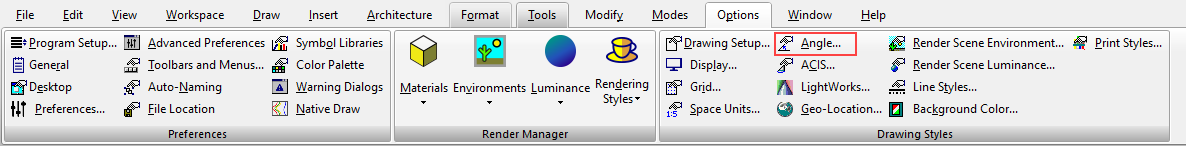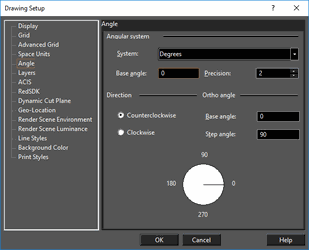# Angle Options

Default UI Menu: Options/Drawing Setup/Angle

Ribbon UI Menu:Controls the measurement and display of angles, as well as control over the Ortho angle.Angular System: Select degrees, degrees-minutes-seconds, grads, radians, or the surveyor system. Base angle: The default base angle is 0 degrees (right quadrant point). You can change this value to start angle measurement from another base angle. Precision: The number of decimal digits. Direction: Choose whether to measure angles clockwise or counterclockwise. Ortho Angle: By default, Ortho lines are 0 and 90 degrees. You can change this by entering new values here. Base angle: Sets the angle from which the Ortho angle is measured. Step angle: The angle to which Ortho constrains lines. If you set the step angle to 15, for example, the line will be constrained to angles 15 degrees apart. The default step angle is 90 degrees.